Home > INT3 > Chapter 11 > Lesson 11.2.4 > Problem11-109

11-109.
1. For each of the following rational expressions, add or subtract, then simplify. Homework Help ✎

2.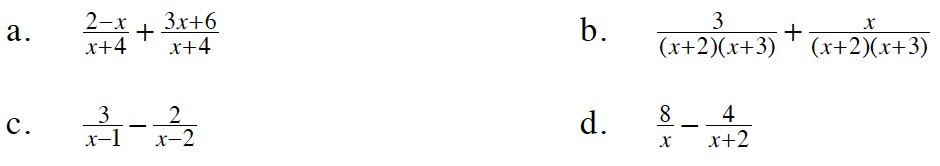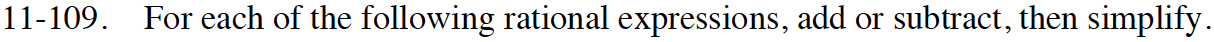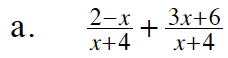The denominators are the same, so just add the numerators.

$\frac{ 2x+8 }{x+4 }$

Factor the numerator and look for a Giant One.

2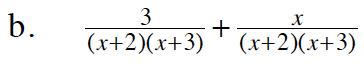See part (a).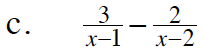The product of the two denominators is the common denominator. Multiply each term by a Giant One to make the denominators the same.

$\left( \frac{x-2}{x-2} \right) \cdot \left( \frac{3}{x-1}\right) -\left( \frac{2}{x-2}\right)·\left( \frac{x-1}{x-1}\right)$

Distribute:

$\frac{(3x-6)}{(x-2)(x-1)}-\frac{(2x -2)}{(x-2)(x-1)}$

Now, subtract. Notice you are subtracting a negative 2 in the second term.

$\frac{x-4}{(x-2)(x-1)}$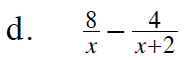See part (c).